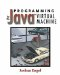# 3.9 Type Conversions

Since most of the arithmetic operations require two arguments of the same type, you will sometimes have to convert numbers of one kind into numbers of another. In Java, a conversion is written with syntax identical to a cast. For example,

` String o = (String) x;         // This is a cast int i = (int) 7.9;             // This is a conversion `

Casts and conversions are actually very different operations. Casting alters the way you are allowed to treat an object without altering the object itself. Conversions have a real effect on the value on the top of the stack. Some conversions may lose information (for example, there's no int that is exactly equivalent to the float 2.71828), but the JVM does its best. See section 4.6 for more about casting.

The internal representation of the float 2 is different from that of the int 2. Although floats have a larger range and can store numbers with a nonintegral component, with large integer values they have to approximate. Take this Java program:

` int i = 2147483647; float f = i; int i2 = (int) f; System.out.println("i = " + i); System.out.println("f = " + f); System.out.println("i2 = " + i2); `

The result is

` i = 2147483647 f = 2.14748365E9 i2 =  2147483648 `

By taking i and converting it to a float, a little information was lost; the difference is about one part in a billion. When you convert back, that difference is enough to cause the value to tick over into negative territory, with the leftmost bit set. This is an extreme case, but it gives you the idea that converting between ints and floats is more than just a matter of what you call the number.

Conversions can affect the height of the stack. The f2d instruction converts a float to a double, which increases the stack height by 1. Converting it back reduces the stack size by 1.

You can convert any numerical type to any other numerical type with a single instruction that converts the top of the stack into some other element. The naming convention for these instructions is x2y, where x is the mnemonic for the type you're converting from and y is the type you're converting to. Table 3.8 summarizes these conversions.

#### 3.9.1 Conversion Example

Following is an example of conversions:

` ldc 1234567890123456789L   ; Push a very long number l2d                        ; As a double: 1.23456789012345677e+18 d2l                        ; Back to long: 1234567890123456768                            ; Some bits were lost on the right l2i                        ; As an int: 2112454912                            ; Lots of bits were lost on the left i2f                        ; As a float: 2.11245e+9 f2l                        ; Back to long: 2112454912 `

##### Table 3.8. Numeric conversions
Mnemonic Stack Result
i2b int int; see section 3.9.2
i2c int int; see section 3.9.2
i2s int int; see section 3.9.2
i2d int double
i2l int long
i2f int float
f2d float double
f2l float long
f2i float int
l2d long double
l2f long float
l2i long int
d2l double long
d2f double float
d2i double int

#### 3.9.2 Widening and Narrowing

Conversion from a number with a smaller range of magnitude (like int to long or long to float) is called widening. Conversely, conversion where there is the possibility of losing information about the magnitude of the number (like long to int or double to long) is called narrowing.

Narrowing operations may lose information about how big the number is. For example, converting the float 3.4028235e38 to long yields -9223372036854775808, a very different number. However, narrowing is not necessarily accompanied by the loss of information. For example, there is an exact representation of the double 1.0 in int: the int 1. Nor is widening necessarily without information loss. For example, converting the int 2147483647 to float produces 2.14748365e9, or 2,147,483,650. The difference is usually small, but it may be significant.

The goal of widening conversions is to produce no change in the magnitude of the number while preserving as much of the precision as possible. With narrowing conversions, some information may be lost, but the nearest representation is found whenever possible.Programming for the Javaв„ў Virtual Machine
ISBN: 0201309726
EAN: 2147483647
Year: 1998
Pages: 158
Authors: Joshua Engel

Similar book on Amazon

flylib.com © 2008-2017.
If you may any questions please contact us: flylib@qtcs.net